# Algebra 1 : How to graph an inequality with a number line

## Example Questions

### Example Question #1 : How To Graph An Inequality With A Number Line

Which line plot corresponds to the inequality below?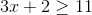Explanation:

A closed circle indicates "greater than or equal to" or "less than or equal to," while and open circle indicates "greater than" or "less than". We can tell from the inequality that our line plot will only have closed circles, as the only symbol is "greater than or equal to."Subtractfrom both sides.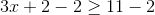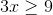Divide both sides by.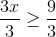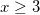Our plot will show a closed circle on, and extend infinitvely in the positive direction.

### Example Question #1 : How To Graph An Inequality With A Number Line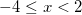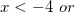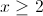This number line includes values that are less thanor greater than or equal to. Note howhas an open circle, whilehas a closed circle.